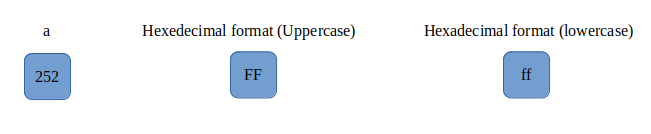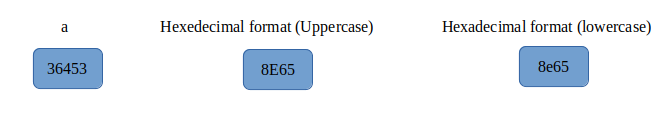# How to Print Hexadecimal in C

In this article, we will see how can we print a number in hexadecimal format using C language, but before that, we will take a look at what this format is all about.

In C language, a value in hexadecimal format is represented by a combination of 16 symbols, out of which 10 symbols are the standard numerical values ranging from 0 to 9 and the rest six are uppercase characters from ‘A’ to ‘F’. Here, the letter ‘A’ represent the numeric value 10, ‘B’ represents 11, ‘C’ represents 12, ‘D’ represents 13, ‘E’ represents 14 and ‘F’ represents 15. These numbers and characters when combined together to represent a value make up the hexadecimal format.

## How to Print Hexadecimal in C

To print a number in hexadecimal format, the format specifier used is ‘%x’ or ‘%X’. Using the ‘%x’ format specifier converts all the letters contained in the hexadecimal representation of a number in lowercase and using the ‘%X’ format converts all the letters in uppercase.

Let us look at some sample programs for better understanding:

Output:Here, the hexadecimal representation of the number 252 is ‘fc’ if the format specifier used is ‘%x’ and ‘FC’ if the format specifier used is ‘%X’. The second print statement is used to insert a line break between the first and third print statements.

Now, let us understand how this conversion actually takes place in the system:

Step 1: Divide the given number by 16 and note the remainder of the operation.

Step 2: Keep repeating step 1 until the quotient becomes 0.

Step 3: Combine the remainders in the reverse order as they are obtained.

For example, in this program, we divide 252 by 16 and obtain 15 as quotient and 12 as remainder. Now, we divide 15 by 16 and obtain 0 as quotient and 15 as remainder. Note that after dividing 15 by 16, the quotient becomes zero. So, we stop our calculation here. The remainders obtained in the two steps are 12 and 15 and their hexadecimal representations are ‘c’ and ‘f’ respectively. We arrange the hexadecimal representation of remainders in the reverse order in which they are obtained. So here, the hexadecimal representation of 252 will be ‘fc’.

Let us take one more example:

Output:The hexadecimal representation of 36453 is 8e65 and it can be calculated in the similar method mentioned above.